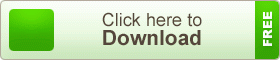# HSC Higher Mathematics 2nd Paper Note 5th Chapter Binomial Expansions

HSC Higher Mathematics 2nd Paper Note 5th Chapter Binomial Expansions. For any power of n, the binomial (a + x) can be expanded. This is particularly useful when x is very much less than so that the first few terms provide a good approximation of the value of the expression. In elementary algebra, the binomial theorem (or binomial expansion) describes the algebraic expansion of powers of a binomial. According to the theorem, it is possible to expand the polynomial (x + y)n into a sum involving terms of the form a xb yc, where the exponents b and c are nonnegative integers with b + c = n, and the coefficient a of each term is a specific positive integer depending on n and b. For example:Special cases of the binomial theorem were known since at least the 4th century B.C. when Greek mathematician Euclid mentioned the special case of the binomial theorem for exponent 2. There is evidence that the binomial theorem for cubes was known by the 6th century in India.

## HSC Higher Mathematics 2nd Paper Note 5th Chapter Binomial ExpansionsThe binomial theorem as such can be found in the work of 11th-century Persian mathematician Al-Karaji, who described the triangular pattern of the binomial coefficients. He also provided a mathematical proof of both the binomial theorem and Pascal’s triangle, using an early form of mathematical induction. The Persian poet and mathematician Omar Khayyam was probably familiar with the formula for higher orders, although many of his mathematical works are lost. The binomial expansions of small degrees were known in the 13th-century mathematical works of Yang Hui and also Chu Shih-Chieh. Yang Hui attributes the method to a much earlier 11th-century text of Jia Xian, although those writings are now also lost.

teachingbd24.com is such a website where you would get all kinds of necessary information regarding educational noes, suggestions and questions’ patterns of school, college, and madrasahs. Particularly you will get here special notes of physics that will be immensely useful to both students and teachers. The builder of the website is Mr. Md. Shah Jamal Who has been serving for 30 years as an Asst. Professor of BAF Shaheen College Dhaka. He expects that this website will meet up all the needs of Bengali version learners /students. He has requested both concerned students and teachers to spread this website home and abroad.